Next: Update Rules for Posterior Up: Nonlinear Independent Component Analysis Previous: Posterior Mean and Variance

# Update Rules

In the previous section we derived all the equations needed for the computation of the cost function. Given the posterior means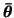and variances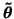and discrete posterior probabilities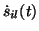, we can compute the cost function which measures the quality of the approximation of the posterior pdf of the unknown variables. Any standard optimisation algorithm could be used for minimising the cost function, but it is sensible to utilise the particular form of the function. Due to lack of space, we shall only outline the update rules but a more detailed description can be found in .

Let us denote C = Cq + Cp, where Cq is the part originating from the expectation of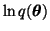and Cp is the part originating from expectation of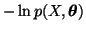. We shall see how it is possible to derive efficient fixed point algorithms for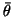and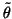assuming that we have computed the gradients of Cp with respect to the current estimates ofand.

Since Cq has a term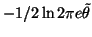for eachwhose posterior is approximated by Gaussian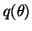, solving for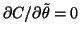yields an update rule for: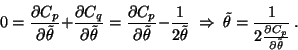(32)

Now suppose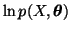is roughly quadratic with respect to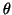:(33)

Then Cp would be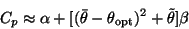(34)

and hence the derivatives with respect toandwould be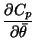=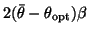(35)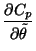=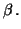(36)

As Cq does not depend on, the optimal value foris evidently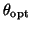and solving for that we obtain an update rule for: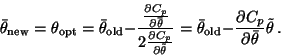(37)

Since this update rule makes a quadratic approximation of the cost function C, it can be viewed as Newton iteration which assumes thatis the only variable which changes because the quadratic approximation does not take into account the cross terms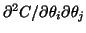. In practice all the weights A and B, for instance, are adapted simultaneously and each weight affects the optimal value of the other weights. In  it is explained how it is possible to compensate for the error which results in the invalid assumption of independent adaptations.Next: Update Rules for Posterior Up: Nonlinear Independent Component Analysis Previous: Posterior Mean and Variance
Harri Lappalainen
2000-03-03